# Verlet modified

The Verlet modified  closure relation for hard sphere fluids, in terms of the cavity correlation function, is (Eq. 3)$Y(r) = \gamma (r) - \left[ \frac{A \gamma^2(r)/2}{1+ B \gamma(r) /2} \right]$

where the radial distribution function is expressed as (Eq. 1)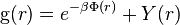${\mathrm g}(r) = e^{-\beta \Phi(r)} + Y(r)$

and where several sets of values are tried for A and B (Note, when A=0 the hyper-netted chain is recovered).

Later Verlet used a Padé (2/1) approximant ( Eq. 6) fitted to obtain the best hard sphere results by minimising the difference between the pressures obtained via the virial and compressibility routes: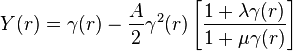$Y(r) = \gamma (r) - \frac{A}{2} \gamma^2(r) \left[ \frac{1+ \lambda \gamma(r)}{1+ \mu \gamma(r)} \right]$

with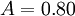$A= 0.80$,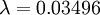$\lambda= 0.03496$ and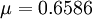$\mu = 0.6586$ where the radial distribution function for hard spheres is written as (Eq. 1)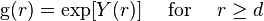${\mathrm g}(r) = \exp[Y(r)] ~~~~ \mathrm{for} ~~~~ r \ge d$

where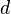$d$ is the hard sphere diameter.